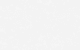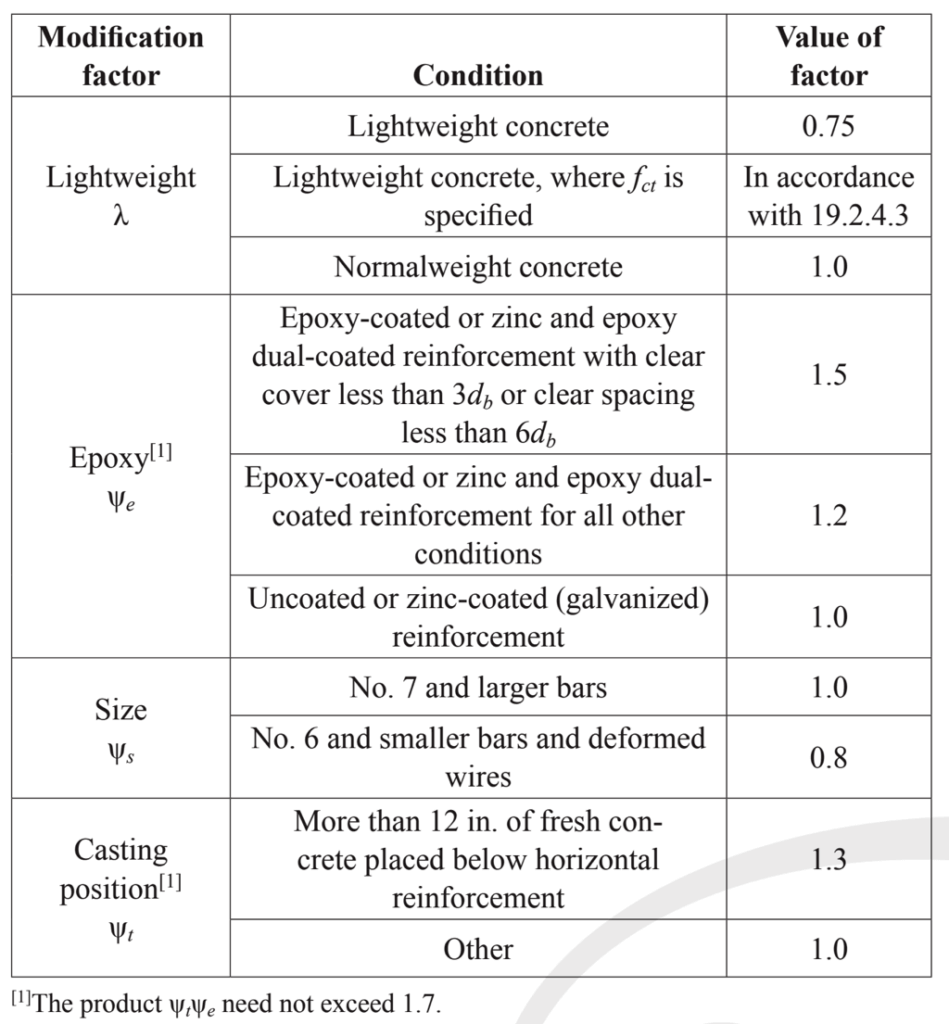# Rebar Development Length Calculator to ACI 318 (US)

### A handy online tool to quickly compute the rebar development length to ACI code in US Customary units.

This online tool lets you calculate the tension and compression development length of a deformed reinforcing bar.

### Input:

Select ACI Formula to be used (see explanation in background section)

Rebar diameter: 0.00 in. (0.00 mm)

Smaller of the distance from center of rebar to nearest concrete surface or one-half of center-to-center spacing between bars being developed
40(Atr/sn), can be conservatively taken as 0

## Result:

Ld = 0.00 x db
Ld = 0.00 in.

#### Tension Lap Splice Length:

L = 0.00 x db (Class B splice)
L = 0.00 in.
For Class A splice, use same as anchorage length.

Ld = 0.00 x db
Ld = 0.00 in.

L = 0.00 x db
L = 0.00 in.

#### Governing factors used:

√(f'c) = 0.00 psi
λ = 1.00
Ψt = 1.00
Ψe = 1.00
Ψt Ψe = 1.00
Ψs = N.A.
(cb + Ktr)/db = N.A.

#### Standard Hook Development Length:

##### 90° Standard HookLdh = 0.00 x db
Ldh = 0.00 in.
r = 0.00 x db = 0.00 in.
c = 0.00 in.
ext = 0.00 in.
h = 0.00 in.

##### 180° Standard HookLdh = 0.00 x db
Ldh = 0.00 in.
r = 0.00 x db = 0.00 in.
c = 0.00 in.
ext = 0.00 in.
h = 0.00 in.

## Background:

### Description

The development length of a rebar is the shortest embedment length required for a reinforcing bar to develop its full yield strength in concrete. This length is governed by the concrete strength and the yield strength of the rebar. Generally, if the concrete is weaker or if the rebar has higher yield strength, longer development length would be required and vice versa. Other factors such as type of rebar coating, spacing, confinement, and position of the rebar also contribute to the determination of development length.

The development length formulas used in this online calculation tool are based on provisions of ACI 318-14. These formulas have not changed much from older versions of the code such as ACI 318-11 or ACI-318-08, hence the calculated output values are compatible with older codes.

### Tension Development Length: Simplified Method

This method uses Section 25.4.2.2 of ACI 318-14 or Section 12.2.2 of ACI 318-11 or older. The code considers the common construction cases.

This provision recognizes that many current practical construction cases use spacing and cover values along with confining reinforcement, such as stirrups or ties, that result in a value of (cb + Ktr)/db of at least 1.5.

Excerpt from ACI 318-14 R25.4.2.2

### Tension Development Length: Detailed Method

This method uses Section 25.4.2.3 of ACI 318-14 or Section 12.2.3 of ACI 318-11 or older. In many situations, you would get smaller tension development lengths by using the detailed method.

In either of the methods, the minimum development length shall not be less than 12 inches.

### Compression Development Length

The compression development length is calculated using Section 25.4.9 of ACI 318-14 or Section 12.3 of ACI 318-11 or older.

The minimum compression development length shall not be less than 8 inches. For compatibility with older ACI codes, Ψr is taken as 1.0. This factor pertains to confinement of the rebar and may be taken as 0.75 subject to certain conditions set in Table 25.4.9.3 of ACI 318-14 (e.g. if ties used are spiral).

### Tension Lap Splice Length

Strictly speaking, tension development length refers to the anchorage length required to develop the full yield strength of the rebar embedded in concrete. On the other hand, splice length is the length required to fully transfer the force from one bar to the adjacent bar.

For Class A lap splices, the required tension lap length is the equal to the tension development length (anchorage length). To classify a lap as Class A, it must comply with onerous conditions defined in the table below.

In the most common cases, the tension lap is considered Class B. In which case, the required lap splice length is 1.3 x development length but not less than 12 inches.

### Compression Lap Splice Length

Compression lap splice length is governed by several conditions as follows:

The minimum compression lap splice length is 8 inches.

### Lapping Bars With Different Diameters

If the bars being lapped have different diameters, the lap splice length is taken as the greater of the development length of larger bar or the splice length of the smaller bar. In general bars which are more than 36mm in diameter shall not be lapped (there are exceptions so refer to code).

These rules apply to both tension or compression lap length computation.

### Development Length Coefficients

The following coefficients (modification factors) are applicable in deriving the development lengths.Excerpt from ACI 318-14 Table 25.4.2.4Modification factors for development of deformed bars and deformed wires in tension

### Development of Standard Hooks in Tension

Deformed bars can be effectively anchored in concrete by curtailing the end of the rebar with a 90° or 180° standard hook allowing the rebar to develop its full strength in tension. ACI 318-14 specifies minimum geometry for standard hooks.

The development length is computed from the maximum of: